Question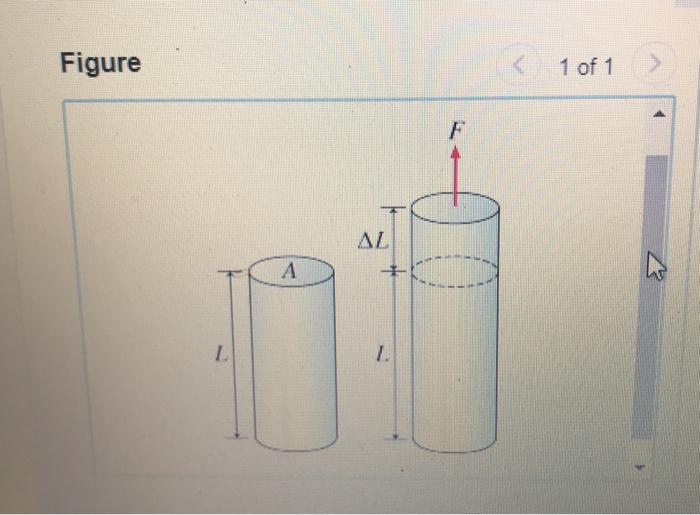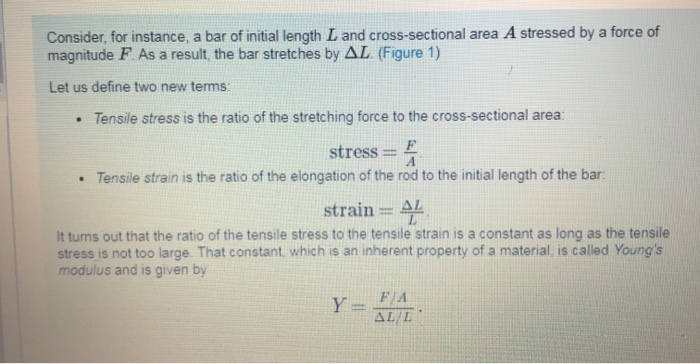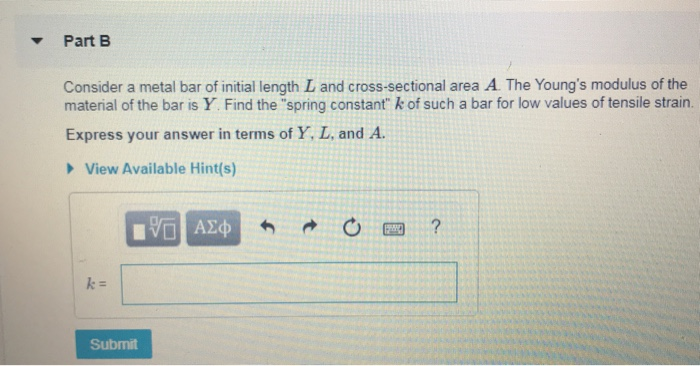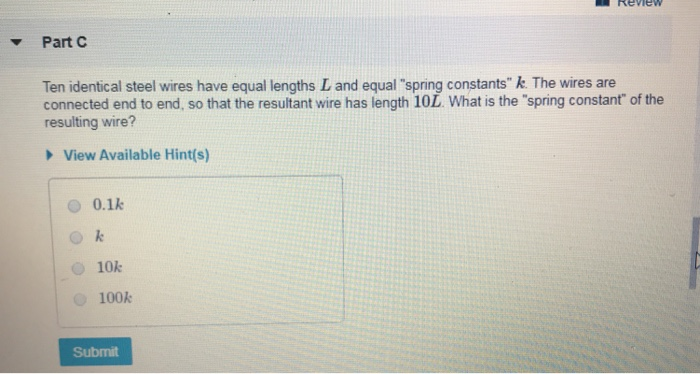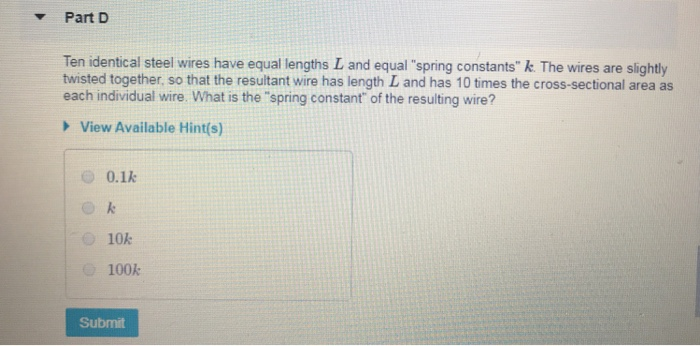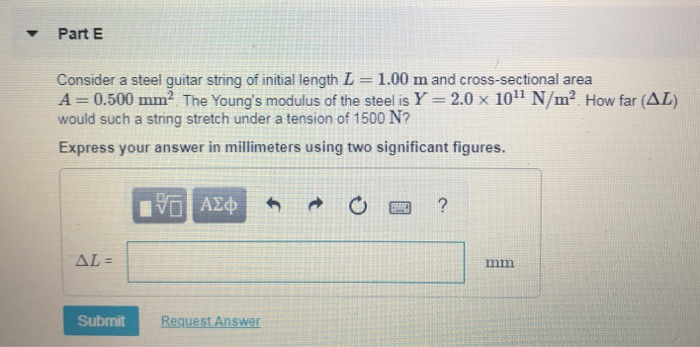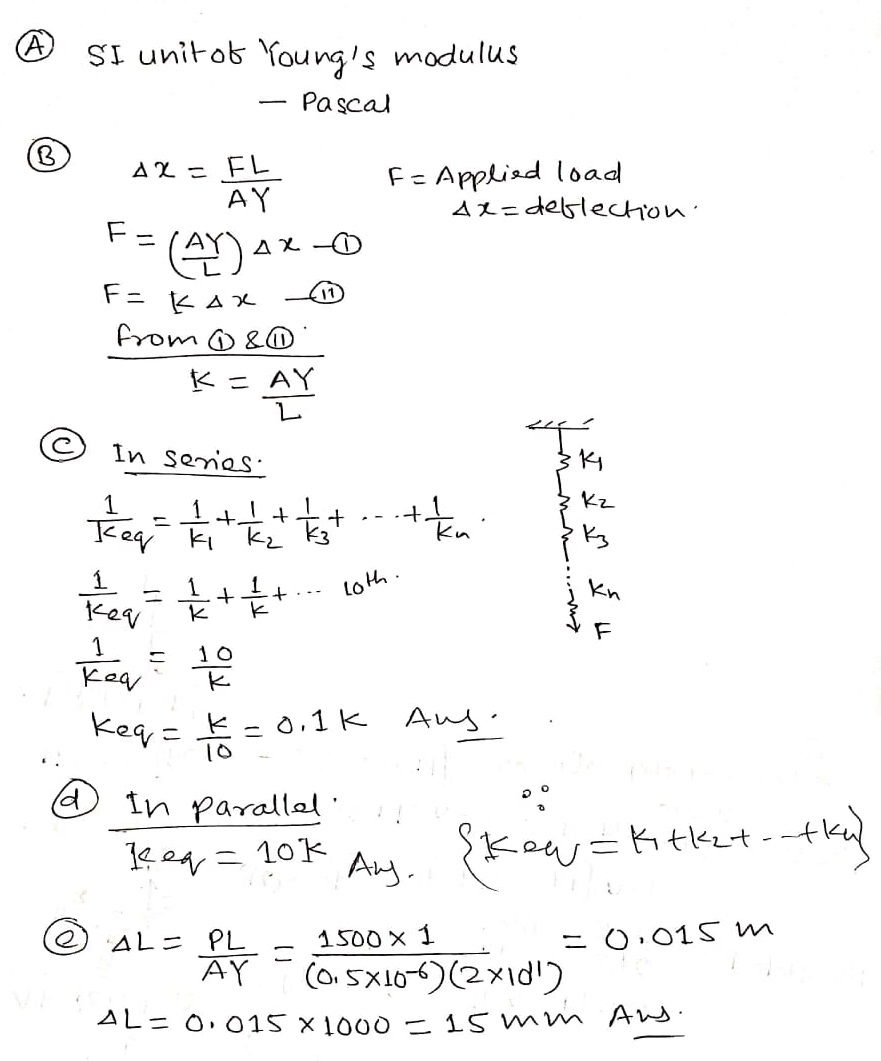#### Earn Coins

Coins can be redeemed for fabulous gifts.

Similar Homework Help Questions
• ### Physics: Youngs modulus, few short related questions, please help, ill rate Lifesaver to some...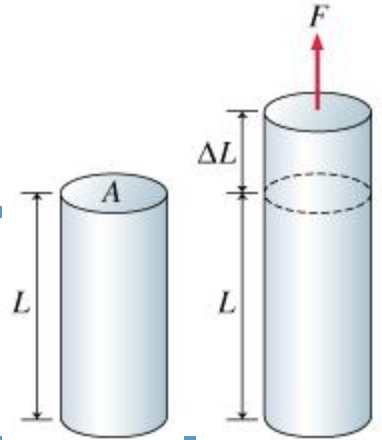Learning Goal:To understand the meaning of Young's modulus and to perform some real-life calculations related to the stretching of steel.Hooke's law states that for springs and other "elastic" objectsF=K(delta x)where F is the magnitude of the stretching force, delta x is the corresponding elongation of the spring from equilibrium, and k is a constant that depends on the geometry and the material of the spring. If the deformations are small enough, most materials, in fact, behave like springs: Their deformation...

• ### Question 1 (10 marks) Consider the bar element shown in Figure 1. Cross sectional area A...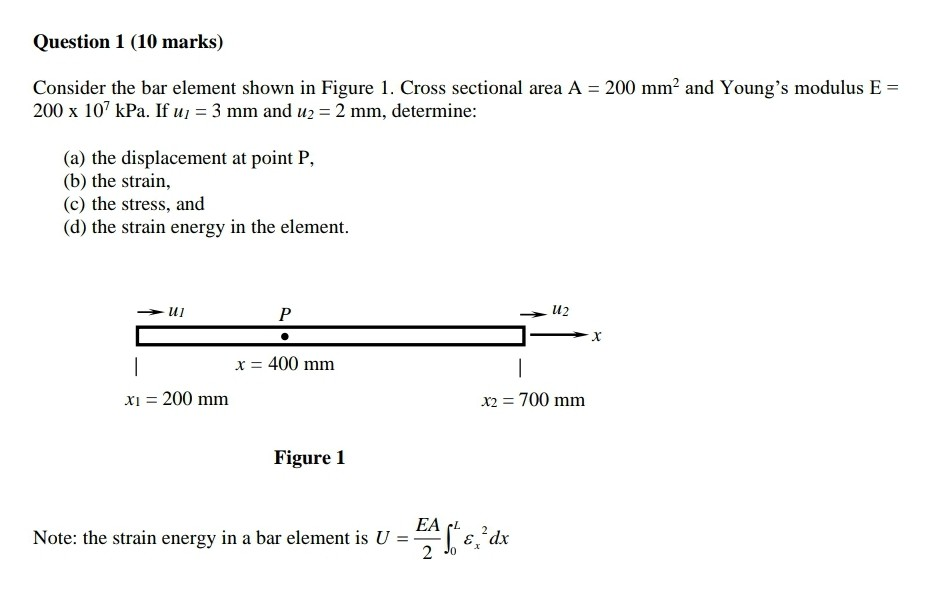Question 1 (10 marks) Consider the bar element shown in Figure 1. Cross sectional area A = 200 mm and Young's modulus E = 200 x 107 kPa. If u = 3 mm and u2 = 2 mm, determine: (a) the displacement at point P, (b) the strain, (c) the stress, and (d) the strain energy in the element. U] U2 x = 400 mm xi = 200 mm x2 = 700 mm Figure 1 EAL 2 Note: the strain...

• ### 3. 2090] Consider a uniform bar of Young's modulus E, cross-sectional area A, moment of inertia d...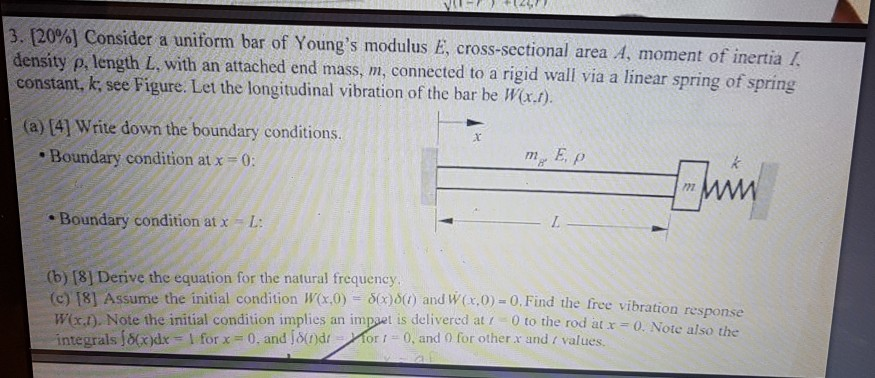3. 2090] Consider a uniform bar of Young's modulus E, cross-sectional area A, moment of inertia density p, length L, with an attached end mass, m, connected to a rigid wall via a linear spring of spring constant, k, see Figure. Let the longitudinal vibration of the bar be Wa.f). (a)  Write down the boundary conditions. m E, p Boundary condition at x 0 Boundary condition at x L (b) [81 Derive the equation for the natural frequency (c)...

• ### 5) Consider a bar shown below. Cross-sectional area Ae = 1.2 in., and Young's modulus E...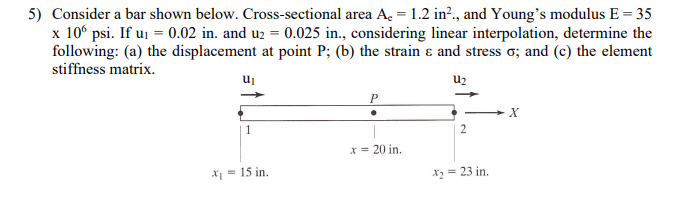5) Consider a bar shown below. Cross-sectional area Ae = 1.2 in., and Young's modulus E = 35 x 106 psi. If ui = 0.02 in, and u2 = 0.025 in., considering linear interpolation, determine the following: (a) the displacement at point P; (b) the strain & and stress o; and (c) the element stiffness matrix. u2 2 x = 20 in. x = 15 in. X = 23 in.

• ### 1. Consider a bar under tension. The bar has length L, rectangular cross-section of sides b...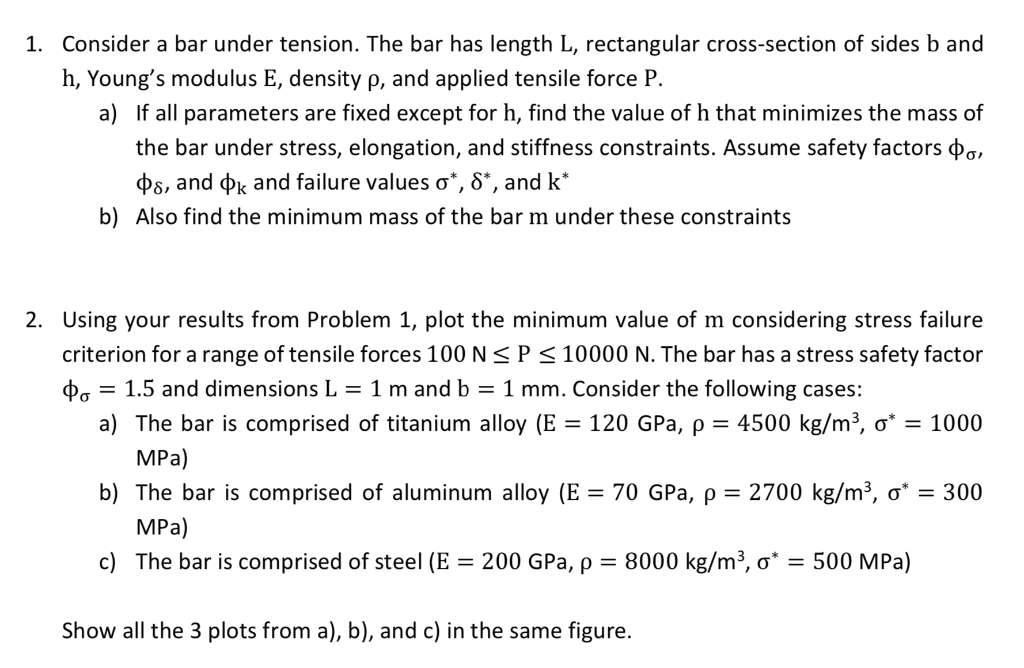1. Consider a bar under tension. The bar has length L, rectangular cross-section of sides b and h, Young's modulus E, density p, and applied tensile force P. a) If all parameters are fixed except for h, find the value of h that minimizes the mass of the bar under stress, elongation, and stiffness constraints. Assume safety factors oo, Os, and Ok and failure values o*, 8*, and k* b) Also find the minimum mass of the bar m under...

• ### 5. EVALUATION I. Create a stress-strain diagram for the measured values in table 1 and identify...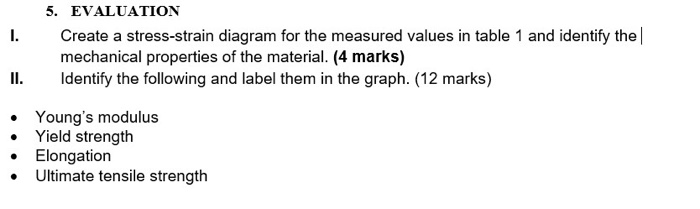5. EVALUATION I. Create a stress-strain diagram for the measured values in table 1 and identify the mechanical properties of the material. (4 marks) II. Identify the following and label them in the graph. (12 marks) • Young's modulus Yield strength Elongation Ultimate tensile strength THEORETICAL BACKGROUND Equations: Cross-sectional Area (A) Modulus of Elasticity (E) Tensile Strength (ST) Percent Elongation (%EL) d? E = Sy Ey Sr Pu А %EL Extension at fracture Gauge Length Where: A: Cross- Sectional Area...

• ### A brass rod with a length of 1.50 m and a cross-sectional area of 2.50 cm2...

A brass rod with a length of 1.50 m and a cross-sectional area of 2.50 cm2 is fastened end to end to a nickel rod with length L and cross-sectional area 0.880 cm2 . The compound rod is subjected to equal and opposite pulls of magnitude 3.46×104 N at its ends. a.Find the length L of the nickel rod if the elongations of the two rods are equal. b.What is the stress in the brass rod? c.What is the stress...

• ### Write down which one of the following statements is false: (A) for a wire of a...

Write down which one of the following statements is false: (A) for a wire of a certain substance and certain cross-sectional area, the spring constant is inversely proportional to natural length (B) Young's modulus is the gradient of stress as a function of strain (C) uids do not have a bulk modulus (D) spring constant is the gradient of load as a function of deformation (E) some substances do not obey Hooke's Law at all

• ### Consider a steel guitar string of initial length 2.00m and cross-sectional area A = 0.500mm^2. The...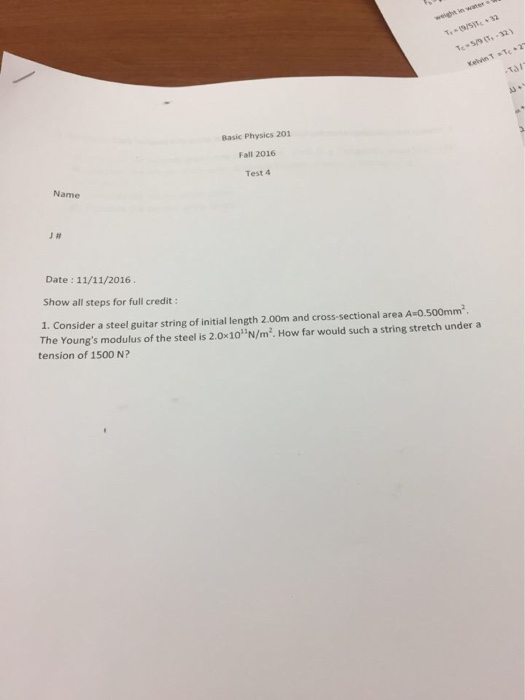Consider a steel guitar string of initial length 2.00m and cross-sectional area A = 0.500mm^2. The Young s modulus of the steel is 2.0 times 10^11 N/m^2. How far would such a string stretch under a tension of 1500 N?

• ### Problem 1 Consider the bar shown below with a cross-sectional area A, 1.2 m2, and Young's...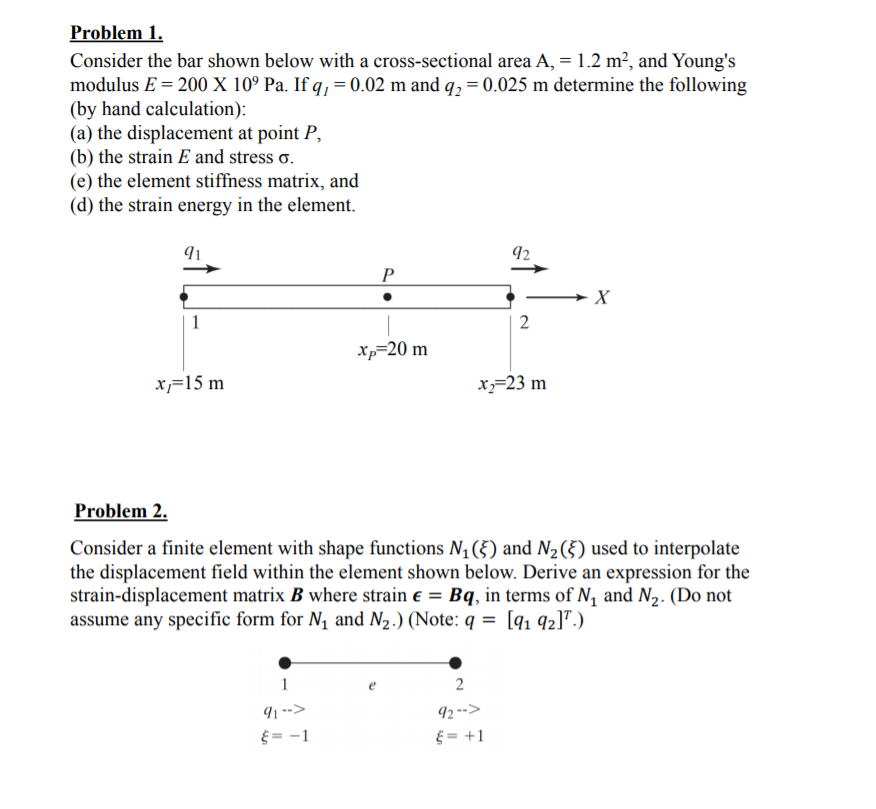Problem 1 Consider the bar shown below with a cross-sectional area A, 1.2 m2, and Young's modulus E-200 X 109 Pa. Ifq,-0.02 m and q,-0.025 m determine the following (by hand calculation) (a) the displacement at point P., (b) the strain E and stress σ (e) the element stiffness matrix, and (d) the strain energy in the element 91 *p 20 m x,-15 m x,-23 m Problem 2. Consider a finite element with shape functions N1) and N2(Š) used to...